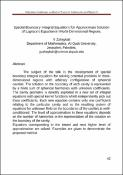## Special Boundary Integral Equations for Approximate Solution of Laplace's Equation in Multi-Dimensional Regions.

 dc.contributor.author Y Zahaykah dc.date.accessioned 2017-05-03T09:37:02Z dc.date.available 2017-05-03T09:37:02Z dc.date.issued 2010-08-02 dc.description.abstract

The subject of the talk is the development of special boundary integral equations for solving potential problems in three-dimensional regions with arbitrary configuration of spherical cavities. The solution on the boundary of each cavity is represented by a finite sum of spherical harmonics with unknown coefficients. The cavity geometry is directly exploited in a new set of integral equations with special kernel functions which independently pick out these coefficients. Each new equation contains only one coefficient relating to the particular cavity and so the resulting system of equations for unknown field on the boundaries of the cavities is well-conditioned. The level of approximation in these equations depends on the number of harmonics in the representation of the solution on the boundary of the cavity.
Equations corresponding to the lowest and next higher level of approximation are solved. Examples are given to demonstrate the proposed method.

en dc.description.abstract

The subject of the talk is the development of special boundary integral equations for solving potential problems in three-dimensional regions with arbitrary configuration of spherical cavities. The solution on the boundary of each cavity is represented by a finite sum of spherical harmonics with unknown coefficients. The cavity geometry is directly exploited in a new set of integral equations with special kernel functions which independently pick out these coefficients. Each new equation contains only one coefficient relating to the particular cavity and so the resulting system of equations for unknown field on the boundaries of the cavities is well-conditioned. The level of approximation in these equations depends on the number of harmonics in the representation of the solution on the boundary of the cavity.
Equations corresponding to the lowest and next higher level of approximation are solved. Examples are given to demonstrate the proposed method.

ar dc.identifier.uri https://hdl.handle.net/20.500.11888/9571 dc.title Special Boundary Integral Equations for Approximate Solution of Laplace's Equation in Multi-Dimensional Regions. en dc.title Special Boundary Integral Equations for Approximate Solution of Laplace's Equation in Multi-Dimensional Regions. ar dc.type Other
##### Original bundle
Now showing 1 - 1 of 1